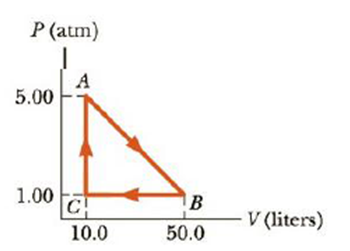Chapter 12, Problem 65AP

Chapter
Section
Textbook Problem

A substance undergoes the cyclic process shown in Figure P12.65. Work output occurs along path AB while work input is required along path BC, and no work is involved in the constant volume process CA. Energy transfers by heat occur during each process involved in the cycle. (a) What is the work output during process AB? (b) How much work input is required during process BC? (c) What is the net energy input Q during this cycle?Figure P12.65

(a)

To determine
The work output during the process AB.

Explanation

Given Info:

The width of rectangle is 40L .

The height of rectangle is 1 atm.

The base of triangle is 40 L.

The height of the triangle is 4 atm.

The area under the graph in a PV diagram is equal in magnitude to the work done on the substance. If the finial volume is greater than the initial volume, then the work done on the substance is negative.

Formula to calculate the area is,

A=(W1×H1)+12(B1×H2)

• W1 is the width of rectangle
• H1 is the height of rectangle
• B1 is the base of triangle
• H2 is the height of triangle

Substitute 40L for width, 1 atm for height for rectangle and 4 atm for height and 40L for base for triangle to find A,

A=(40L){[1atm(1

(b)

To determine
The work input required during the process BC.

(c)

To determine
The net energy input during the cycle.

Still sussing out bartleby?

Check out a sample textbook solution.

See a sample solution

The Solution to Your Study Problems

Bartleby provides explanations to thousands of textbook problems written by our experts, many with advanced degrees!

Get Started